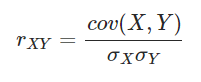# Artificial Intelligence Interview Questions

#### Q1. What is AI?

AI is a branch of computer science that stresses and finds a way of creating an intelligent machine that has the ability to work, think and reacts like humans.

#### Q2. How aware you think you are in terms of using AI enabled devices and services?

Like I said that AI is everywhere and currently has a deep impact on our surroundings, we can see AI touch in the below listed things:

• Smartphones
• Smart Cars and Drones
• Social Media Feeds
• Media Players
• Video Games and many more Areas

#### Q3. What do you think are the areas where AI has a great impact?

AI has a great influence on numerous areas.

At present it is:

• Computing Field
• Speech Recognition
• Bioinformatics
• Humanoid Robots
• Space and Aeronautics
• Weather Forecasting

#### Q4. Which programming languages are preferred for AI?

This is the common Artificial Intelligence interview questions asked in an interview. The programming language which is preferred for AI are Python, R, Lisp, Prolog, and Java.

#### Q5. Narrate the formula for the coefficient for logistic regression?

The logistic regression is given by:

• πi=Pr(Yi=1|Xi=xi)=exp(β0+β1xi)1+exp(β0+β1xi)

#### Q6. What do you mean by overfitting and underfitting algorithms?

• Overfitting and Underfitting are responsible for poor performance.
• Overfitting gives a good performance on the trained data, poor generalization to other data.
• Underfitting gives poor performance on the training data and good generalization to other data.

#### Q7. Explain tree topology?

As the name suggests “Tree” topology has several connected elements arranges like the branches of a tree. The structure has at least three specific levels in the hierarchy. These are scalable and accessible while troubleshooting and are so preferred. A common drawback in this topology is the hindrance or malfunctioning of the primary node.

#### Q8. Narrate some of the branches of AI?

There are some branches of AI are as follow:

• Automatic Programming
• Constraint Satisfaction
• Bayesian Networks
• Knowledge Representations
• Machine Learning
• Natural Language Processing (NLP)
• Neural Networks
• Robotics
• Speech Recognition

#### Q9. Explain karl pearson’s coefficient of correlation?

Karl Pearson’s correlation coefficient is a measure of the strength of a linear association between two variables. It is denoted by r or rxy (where x and y being the two variables involved). This method of correlation draws a line of best fit through the data of two variables.
The value of the Pearson correlation coefficient (r) indicates how far away all these data points are to this line of best fit.

Formula:Where,

* cov(X, Y): is the covariance between X and Y.

#### Q10. How to select the best hyperparameters in a tree-based model?

There are two best Hyperparameter in a tree-based model:

• Measure the performance over training data.
• Measure the performance over validation data.

We have to consider the validation result while comparing with the test results, so the answer is B.

#### Q11. What did you know about the Agent in AI? Tell me all the relevant details of it?

In my understanding first, we have an agent formula.Agent = Architecture + Agent program

Let’s see a practical example of an agent. In our human body, three are eyes, ears which act as a sensor and also the legs and hands and other body parts that Acta as actuators.

So, an AI system is composed of an agent and its environment. All the time an agent acts in its environment.

So anything can be considered as an agent if it has:

• Perceiving the environment using its sensors.
• Acting or behaving upon related environment using actuators.
• #### 12. List down the techniques or algorithms mostly used in AI?

In general, there are certain algorithms that are mostly used, or we can say that they are the first one to approach to understand the complex scenarios.

#### Q13. Explain the objective and related terminology used in the search algorithms of AI?

This is the most popular Artificial Intelligence Interview Questions asked in an interview. Searching is the universal techniques used in AI problem techniques. This algorithm is used to search a particular position.

Every search terminology has some components.

• Problem space: This is the environment in which the search takes place.
• Problem Instance: It’s a result of the Initial State + Goal state.
• Problem Space Graph: This is used to represent a problem state.
• The depth of a problem: Here we can define the length of the shortest path.
• Space Complexity: We can calculate this by the maximum number of nodes that are stored in memory.
• Time Complexity: It is defined as the maximum number of nodes that are created.
• Admissibility: This is the property of the algorithms that are used to find the optimal solutions.
• Branching Factors: This can be calculated by the average number of child nodes in the problem space graph.
• Depth: It is the length of the shortest path from inception to the goal state.

Here are some of the search algorithms:

#### Q14. List down some of the best AI software platforms?

Following are the best AI software platforms:

• Tensor Flow
• Azure Machine Learning
• Ayasdi
• Playment
• Salesforce Einstein
• Cloud Machine Learning

Source : Image -cio.com

Content : https://www.educba.com/